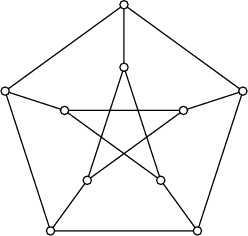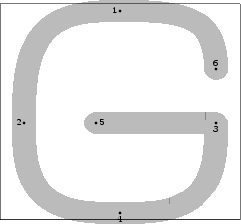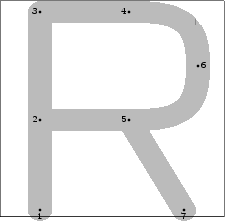# Introduction

METAGRAPH is a small set of macros that help in drawing (un)directed graphs with METAPOST and the boxes package. The basic idea is providing low-impact definitions that produce automatically (labelled) nodes/vertices and (labelled) edges/arcs that connect correctly boxed items. Moreover, circular boxes with fixed radius are made available to produce more aesthetically pleasant graphs.

# ﻿Usage

Just include metagraph.mp in your source files. Full documentation is provided with the package.

# ﻿Examples% Petersen's graph

for i = 0 upto 4:
node.x[i]; node.y[i];
x[i].c = up scaled 60 rotated (360i/5);
y[i].c = up scaled 30 rotated (360i/5);
endfor;

for i = 0 upto 4: drawboxed(x[i],y[i]); endfor;

for i = 0 upto 4:
draw arc(x[i])(--)(x[(i+1)mod 5]);
draw arc(y[i])(--)(y[(i+2)mod 5]);
draw arc(x[i])(--)(y[i]);
endfor;% A bells-and-whistles example
prologues := 2;
x1c = x0c + (2cm,0);
drawboxed(x0, x1);
drawarrow larc(x0)(--)(x1)(btex $a$ etex);
drawarrow larc.bot(x0)({down}..)(x1)(btex $b$ etex);
drawarrow lloop(x0, left, btex $\delta$ etex);
drawarrow lloop(x1, right, btex $\sqrt2$ etex) dashed evenly;
drawarrow lloop.llft(x1, 2right, btex $\gamma$ etex) dashed evenly;


# ﻿The logo

To be able to write METAGRAPH using the METAFONT logo font, a few new letters had to be created. We hope Don Knuth and John Hobby forgive us:beginlogochar("G",15);
x1=x4=.5w; top y1=h+o; bot y4=-o;
x2=w-x3=good.x(1.5u+s); y2=y3=y5=barheight;
x5=x3-.5w; draw z3--z5;
x6=x3; y6=y3+h/4;
%x5=.5[w-leftstemloc,lft w]; x6=x5-.5w; y6=y5=barheight;
super_half(2,1,6); super_half(2,4,3);
labels(1,2,3,4,5,6); endchar;

beginlogochar("H",15);
x1=x2=x3=leftstemloc;
x4=x5=x6=w-x1+ho;
top y1=top y4=h+o;
y2=y5=barheight;
bot y3=bot y6=-o;
draw z1--z3; draw z4--z6; draw z2--z5;
labels(1,2,3,4,5,6); endchar;

beginlogochar("R",14);
x1=x2=x3=leftstemloc;
x4=x5=.618[x1,w-x1];
x7=w-x2; y1=y7;
y2=y5; y3=y4; bot y1=-o;
top y3=h; y2=barheight;
draw z1--z3--z4; draw z2--z5; draw z5--z7;
x6=.5[w-x1,lft w]; y6=.5[y4,y5];
super_crescent(4,6,5);
labels(1,2,3,4,5,6,7); endchar;